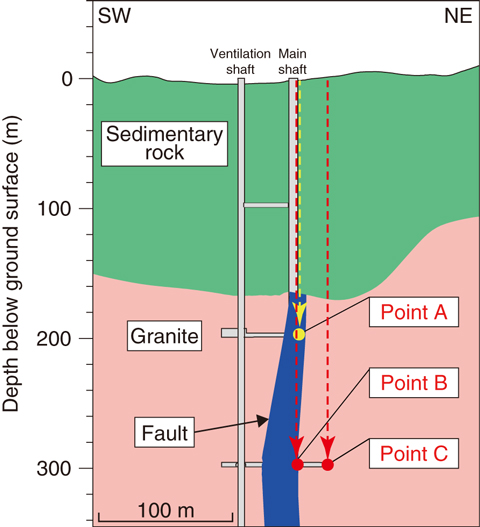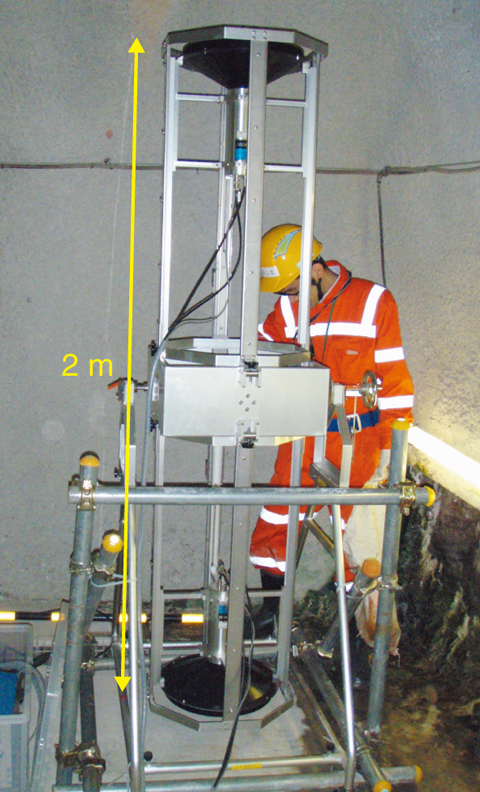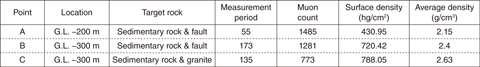# 8-5　Estimation of Underground Density Structure

## －Development of a Technique to Estimate Underground Density Structure using Cosmic-Ray Muons－Fig.8-13 Geological model (cross-section) showing the distribution of rock units and geological structures in the Mizunami Underground Research Laboratory with measurement points for cosmic-ray muons

We measured upwards-directed cosmic-ray muons at each measurement point.Fig.8-14 Photograph of the experimental-muon telescope

The telescope is equipped with two plastic scintillators 35 cm in diameter and 3 cm thick to detect simultaneous transmission of muons.

Table 8-1 Measurement results at points A, B, and C

Surface density indicates the weight per unit area. The densities of sedimentary rock, granite, and fault are based on the calculated surface densities and transmission distances of muons in each geological unit, which in turn coincide with the thicknesses of sedimentary rock, granite, and fault, respectively.This Picture(86KB)

Muons are elementary particles; although they are highly transmittable through materials, the number of transmittable muons decreases in proportion to material density and transmission distance. Thus, muons have been widely utilized to estimate internal structures such as those of active volcanos and the TEPCO’s Fukushima Daiichi NPS.

To assess the safety of geological disposal of highly radioactive waste, the distribution of major geological structures such as fault needs to be confirmed. Three rock units—granite, fault, and sedimentary rock—are distributed in the Mizunami Underground Research Laboratory (Fig.8-13). We examined whether we could estimate the densities of these three rock units by means of muon measurements.

Muons were measured at three points, considering the fault distribution (Fig.8-13) with a muon telescope shown in Fig.8-14. The telescope was equipped with two plastic scintillators. We counted the simultaneous transmission of muons with two scintillators (Table 8-1) and calculated the surface densities from the muon counts and transmission distances of each measurement point based on an equation obtained from previous literature (Table 8-1).

The surface density indicates weight per unit area. The weight is the sum of the products of the density and thickness of each rock unit (for example, the weight at measurement point A is sum of the product of density and thickness of the sedimentary rock and fault). Because the thickness of each rock unit was are already known (Fig.8-13), the densities of these units can be calculated by the surface densities of the three measurement points. Consequently, we obtained densities of 3.38 g/cm3 for granite, 2.88 g/cm3 for fault, and 1.99 g/cm3 for sedimentary rock, respectively.

The obtained densities are much higher than the previously measured values. The estimated density of granite obtained by muon observation is approximately 30% larger than the previously measured density (c. 2.6 g/cm3). If the density of granite is assumed to be 2.6 g/cm3, then the re-calculated densities of fault and sedimentary rock are 2.2 and 1.5 g/cm3, respectively. The calculated densities accord with the previously measured densities.

We concluded that cosmic-ray muons have a high potential for detecting geological structures with different densities by confirming the ratio of the densities amongst the three rock units, although it is not clear why the density values obtained were obviously higher than previously obtained values. We expect muons to be applicable to the investigation of geological structures such as fault in the future.

| | | | |